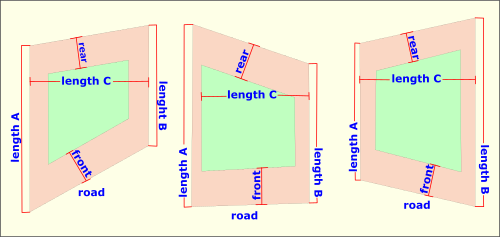Spike's Calculators

# Lot and Building Envelope Square Footage

This calculator calculates the square footage of a lot where the side property lines run parallel with each other. In addition it will calculate the building envelope based on building setbacks.##### For the calculation to be correct you have to match the lengths a, b and c to their location in the image.
Length c ft in
Length a ft in
Length b ft in
Front Set Back ft
Rear Set Back ft
Side Set Back ft

#### Results:

 Square Footage of Lot ft² Square Footage of Building Envelope ft² Perimeter of Building Lot ft

#### The Calculation

1. length c
2. length a
3. length b
4. front setback
5. rear setback
6. side setback

This second calculator can be used for a building lot where the front and back property lines run parallel with each other and it also calculates the square footage of the building envelope.##### For the calculation to be correct you have to match the lengths a, b and c to their location in the image.
Length A ft in
Length B ft in
Length C ft in
Front Set Back ft
Rear Set Back ft
Side Set Back ft

#### Results:

 Square Footage of Lot ft² Square Footage of Building Envelope ft² Perimeter of Building Lot ft

#### The Calculation

1. length a
2. length b
3. length c
4. front setback
5. rear setback
6. side setback